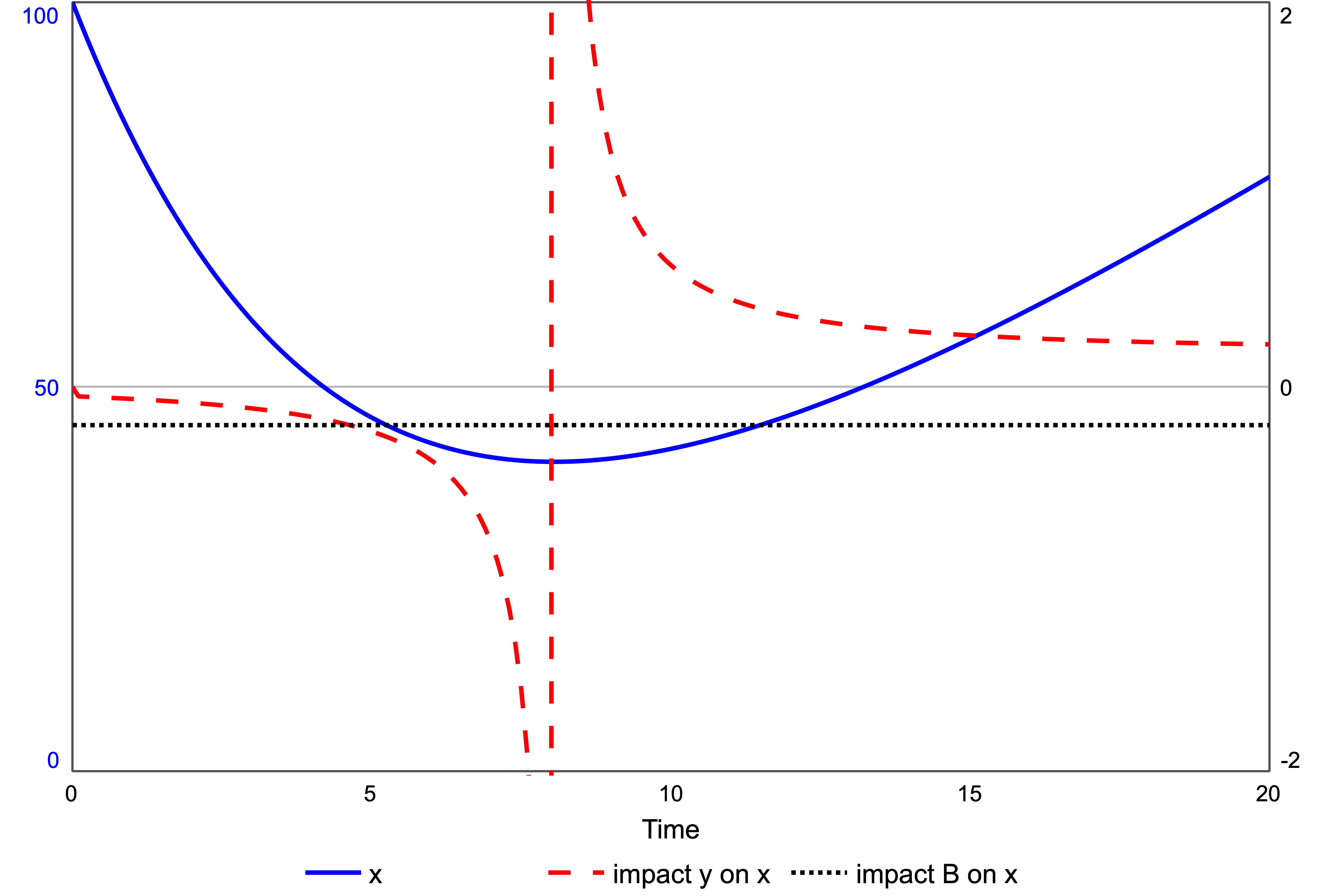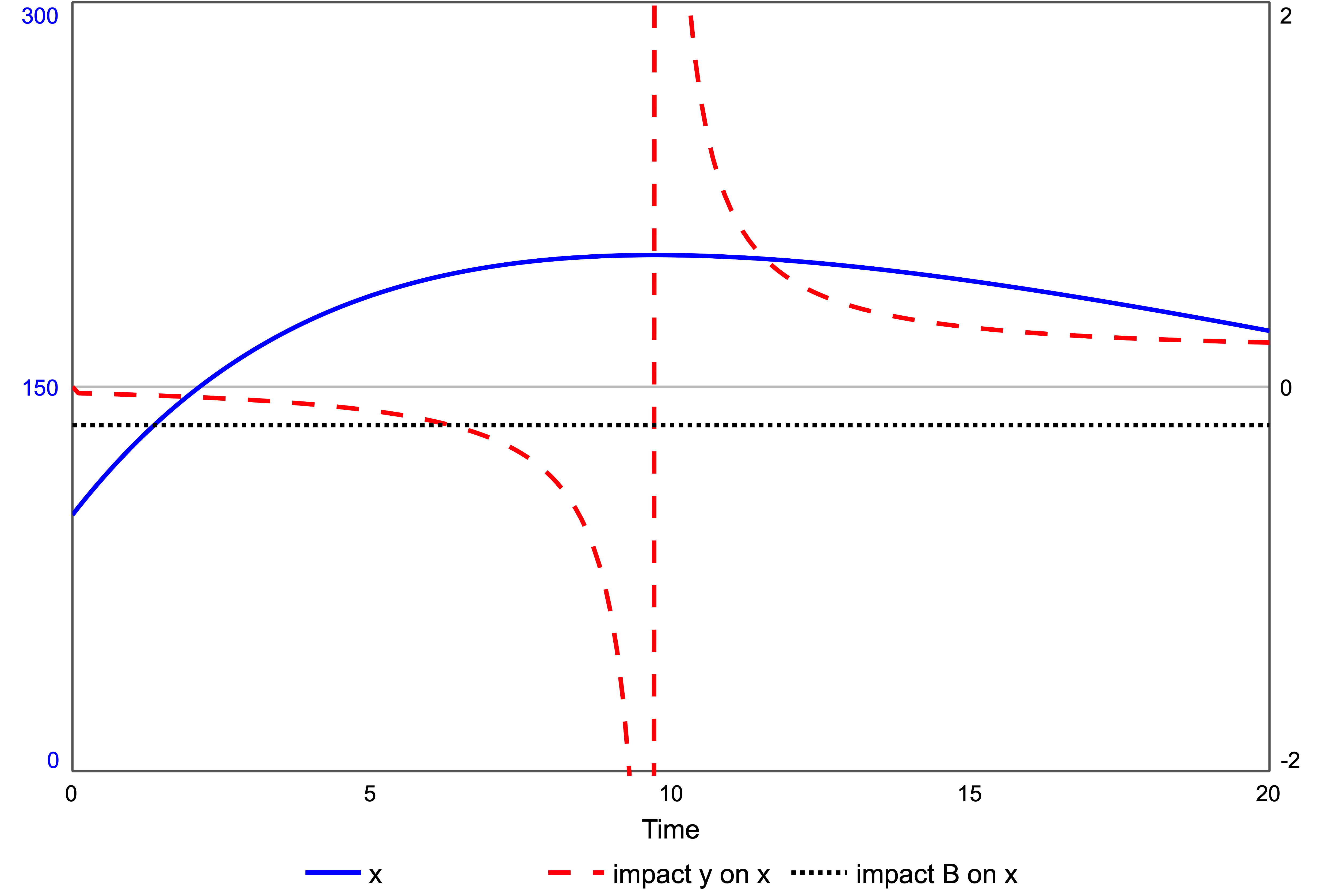# Force and Impact

In mechanics, force produces acceleration in an object. The same concept can be used in system dynamics to describe how any time-varying signal can cause acceleration in a stock – a dynamic variable. That signal may be exogenous or come from another stock. In the Newtonian Interpretive Framework, the effect of the force is measured by impact, the ratio of the acceleration of the stock to its rate of change – net flow; the equivalent of velocity. If x is a stock, the impact of all forces on the stock gives:

Thus, force and impact have different sign conventions depending on the net flow dx/dt.

To compare these differences, imagine you are sitting at the origin where there is a positive force on nearby objects – repelling force. If the object is at rest, the force accelerates it, causing it to move away. If the object is already moving away, the force still accelerates it, moving it away faster. The positive force produces positive acceleration. From the impact equation (1), the impact of the force on the stock is positive as both numerator and denominator are positive.

But if an object is moving toward you, the positive force tries to slow the object in order to stop it and send it away again. Thus, the object decelerates. A positive force now has a negative effect on the object. From equation (1) the impact of the force on the object is negative as the denominator dx/dt is negative for an object move toward the origin.

Therefore, a positive force may have a positive impact, or a negative impact, depending on whether the object is moving away, or moving towards, the origin. Impact picks up the effect of the force on the object.

The same comparison between force and impact applies in system dynamics. Consider a stock y exerting a positive force on stock x, figure1. A positive force has g > 0. Stock x is also subject to a negative force, the balancing feedback loop B, a resistive force.

The flow g is set to a constant, g = 1, so that y increases uniformly, starting from zero. Thus, the force of y on x is constant and positive, equal to one. With x starting at 100, the force from the balancing loop is much larger than that of y; thus, x declines, figure 2. Thus, dx/dt < 0 and the impact of y on x is negative, the dashed line in figure 2. A positive force, but a negative impact. The impact of loop B is also negative as the loop is balancing, dotted line figure 2.Figure 2. Stock x, left axis. The impacts of y and loop B on x. Positive force, g = 1.

Initially, loop B exerts the strongest force on x, indicated by its impact having greater magnitude that that from y. At time 4.5, the impact of y exceeds the balancing loop. It is this exogenous force that is responsible for bringing the stock to rest, a momentary equilibrium, at time 8.0 .

After this minimum point, the stock x starts to increase again, driven by the force from y now acting as an accelerator. A positive force with a positive impact, indicated in figure 2 by the dashed line jumping from negative to positive. Impact from y on x is momentarily infinite at x‘s minimum, as dx/dt = 0.

From time = 8 onward, the balancing loop B is resisting the positive force from y, but it remains smaller. As time tends to infinity, the impact of y on x tends to the absolute value of the impact of B, with x tending to uniform increase.

The situation is reversed if the force is negative – an attracting force. In the model of figure 1, a negative force occurs if g < 0 . Let g = -1 with y starting at 50. Stock x increases to a maximum, then declines, figure 3.Figure 3. Stock x, left axis. The impacts of y and loop B on x. Positive force, g = -1.

Figure 3 shows the impact of y on x is the same as that for the positive force, figure 2. Up to the maximum point, the negative force has a negative impact, slowing the growth of x. After the maximum, the negative force has a positive impact, accelerating the decline of x.

An advantage of measuring the force by impact is that the sign of the impact matches the type of behaviour, plus mean acceleration, minus meaning deceleration. It follows that impact is related to the curvature in the graph of the stock. A further advantage of impact is that if the force comes from a first-order loop, impact is positive, with a negative sign for balancing loops. Thus, impact is a natural way of measuring force in system dynamics.

For more information read Hayward J. & Roach P.A. (2017). Newton’s Laws as an Interpretive Framework in System Dynamics. System Dynamics Review, 33(3-4), 183-218.  DOI: 10.1002/sdr.1586.

 Although a first-order balancing loop can bring a stock to rest (equilibrium), it only does so in the limit as time tends to infinity. It requires an exogenous effect, or a higher-order loop, to bring a stock to rest in a finite time.

 The force from y to x can also be made negative by altering the connection between y and the flow into x such that a rising y gives falling flow, e.g. f1 = 1/y.